Probability - NCERT Questions
Q 1.

Complete the following statements:
(i) Probability of an event E + Probability of the event 'not E' = ................. .
(ii) The probability of an event that cannot happen is ................. . Such an event is called ................. .
(iii) The probability of an event that is certain to happen is ................. . Such an event is called ................. .
(iv) The sum of the probabilities of all the elementary events of an experiment is ................. .
(v) The probability of an event is greater than or equal to ................. and less than or equal to ................. .

SOLUTION:

Soln. : (i) Probability of an event E + Probability of the event 'not E' = 1.
(ii) The probability of an event that cannot happen is 0. Such an event is called impossible
event.
(iii) The probability of an event that is certain to happen is 1. Such an event is called sure or
certain event.
(iv) The sum of the probabilities of all the elementary events of an experiment is 1.
(v) The probability of an event is greater than or equal to 0 and less than or equal to 1.

Q 2.

Which of the following experiments have equally likely outcomes? Explain.
(i) A driver attempts to start a car. The car starts or does not start.
(ii) A player attempts to shoot a basketball. She/he shoots or misses the shot.
(iv) A baby is born. It is a boy or a girl.

SOLUTION:

Soln. : (i) Since he may or may not start the car thus the outcomes are not equally likely.
(ii) The player may shoot or miss the shot.
∴ The outcome are not equally likely.
(iii) In advance it is known that the answer is to be either right or wrong.
∴ The outcomes right or wrong are equally likely to occur.
(iv) In advance it is known that newly born baby has to be either a boy or a girl.
∴ The outcomes either a boy or a girl are equally likely to occur.

Q 3.

Why is tossing a coin considered to be a fair way of deciding which team should get the ball at the beginning of a football game?

SOLUTION:

Soln. : Since on tossing a coin, the outcomes 'head' and 'tail' are equally likely, the result of tossing a coin is completely unpredictable and so it is a fair way.

Q 4.

Which of the following cannot be the probability of an event?
(A)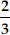(B) -1.5
(C) 15%
(D) 0.7

SOLUTION:

Soln. : (B) Since, the probability of an event cannot be negative,
∴ -1.5 cannot be the probability of an event.

Q 5.

If P(E) = 0.05, what is the probability of 'not E'?

SOLUTION:

Soln. :P(E) + P(not E) = 1
∴ 0.05 + P(not E) = 1 ⇒ P(not E) = 0.95
Thus, probability of 'not E' = 0.95.

Q 6.

A bag contains lemon flavoured candies only. Malini takes out one candy without looking into the bag. What is the probability that she takes out
(i) an orange flavoured candy?
(ii) a lemon flavoured candy?

SOLUTION:

Soln. : (i) Since there are lemon flavoured candies only in the bag.
∴ Taking out orange flavoured candy is not possible.
⇒ Probability of taking out an orange flavoured candy = 0.
(ii) Also probability of taking out a lemon flavoured candy = 1.

Q 7.

It is given that in a group of 3 students, the probability of 2 students not having the same birthday is 0.992. What is the probability that the 2 students have the same birthday?

SOLUTION:

Soln. : Let the probability of 2 students having same birthday = P(SB)
And the probability of 2 students not having the same birthday = P(NSB)
P(SB) + P(NSB) = 1
P(SB) + 0.992 = 1 ⇒ P(SB) = 1 - 0.992 = 0.008

Q 8.

A bag contains 3 red balls and 5 black balls. A ball is drawn at random from the bag. What is the probability that the ball drawn is
(i) red? (ii) not red?

SOLUTION:

Soln. : Total number of balls = 3 + 5 = 8
∴ Number of possible outcomes = 8
(i) ∵ There are 3 red balls.
∴ Number of favourable outcomes = 3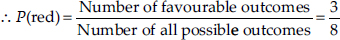(ii) Probability of the ball drawn which is not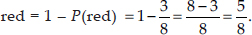Q 9.

A box contains 5 red marbles, 8 white marbles and 4 green marbles. One marble is taken out of the box at random. What is the probability that the marble taken out will be (i) red? and (ii) white? (iii) not green?

SOLUTION:

Soln. : Total number of marbles = 5 + 8 + 4 = 17
∴ Number of all possible outcomes = 17
(i) ∵ Number of red marbles = 5
∴ Number of favourable outcomes = 5
∴ Probability of red marbles,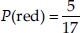(ii) ∵ Number of white marbles = 8
∴ Probability of white marbles,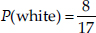(iii) ∵ Number of green marbles = 4
∴ Number of marbles which are not green
= 17 - 4 =13
i.e., Favourable outcomes = 13
∴ Probability of marbles 'not green',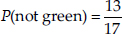Q 10.

A piggy bank contains hundred 50p coins, fifty ₹ 1 coins, twenty ₹ 2 coins and ten ₹ 5 coins. If it is equally likely that one of the coins will fall out when the bank is turned upside down, what is the probability that the coin (i) will be a 50p coin? and (ii) will not be ₹ 5 coin?

SOLUTION:

Soln. : Number of:
50 p coins = 100, ₹ 1 coins = 50
₹ 2 coins = 20, ₹ 5 coins = 10
Total number of coins
= 100 + 50 + 20 + 10 = 180
∴ Total possible outcomes = 180
(i) For a 50 p coin:>
Favourable events = 100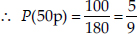(ii) For not a ₹ 5 coin:
∵ Number of ₹ 5 coins = 10
∴ Number of 'not ₹ 5' coins = 180 - 10 = 170
⇒ Favourable outcomes = 170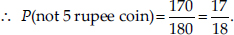Q 11.

Gopi buys a fish from a shop for his aquarium. The shopkeeper takes out one fish at random from a tank containing 5 male fish and 8 female fish. What is the probability that the fish taken out is a male fish?

SOLUTION:

Soln. : Number of male fishes = 5
Number of female fishes = 8
∴ Total number of fishes = 5 + 8 = 13
⇒ Total number of outcomes = 13
For a male fish:
Number of favourable outcomes = 5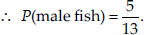Q 12.

A game of chance consists of spinning an arrow which comes to rest pointing at one of the numbers 1, 2, 3, 4, 5, 6, 7, 8 (see figure), and these are equally likely outcomes. What is the probability that it will point at
(i) 8?
(ii) an odd number?
(iii) a number greater than 2?
(iv) a number less than 9?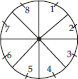SOLUTION:

Soln. : Total number marked = 8
∴ Total number of possible outcomes = 8
(i) When pointer points at 8:
Number of favourable outcomes = 1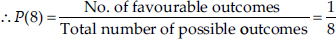(ii) When pointer points at an odd number:
∵ Odd numbers are 1, 3, 5 and 7
∴ Number of odd numbers from 1 to 8 = 4
⇒ Number of favourable outcomes = 4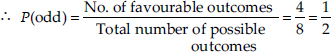(iii) When pointer points at a number greater than 2:
[∵ The numbers 3, 4, 5, 6, 7 and 8 are greater than 2]
∴ Number of numbers greater than 2 = 6
⇒ Number of favourable outcomes = 6
P(greater than 2)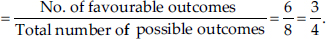(iv) When pointer points a number less than 9:
Q The numbers 1, 2, 3, 4, 5, 6, 7 and 8 are less than 9.
∴ Number of numbers less than 9 = 8
∴ Number of favourable outcomes = 8
P(less than 9)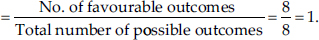Q 13.

A die is thrown once. Find the probability of getting :
(i) a prime number
(ii) a number lying between 2 and 6
(iii) an odd number.

SOLUTION:

Soln. : Since, numbers on a die are 1, 2, 3, 4, 5 and 6.
∴ Number of total possible outcomes = 6
(i) Since 2, 3 and 5 are prime numbers,
∴ Favourable outcomes = 3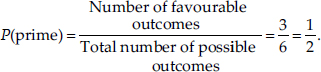(ii) Since the numbers between 2 and 6 are 3, 4 and 5
∴ Favourable outcomes = 3
∴ Required probability =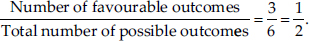(iii) Since 1, 3 and 5 are odd numbers.
⇒ Favourable outcomes = 3
Required probability =Q 14.

One card is drawn from a well-shuffled deck of 52 cards. Find the probability of getting:
(i) a king of red colour
(ii) a face card
(iii) a red face card
(iv) the jack of hearts
(vi) the queen of diamonds

SOLUTION:

Soln. : Number of cards in deck = 52
∴ Total number of possible outcomes = 52
(i) ∵ Number of red colour kings = 2
[∵ King of diamond and heart is red]
∴ Number of favourable outcomes = 2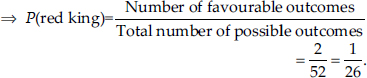(ii) For a face card:
∵ 4 kings, 4 queens and 4 jacks are face cards
∴ Number of face cards = 12
⇒ Number of favourable outcomes = 12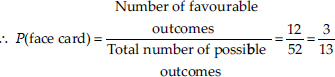(iii) Since, cards of diamond and heart are red
∴ There are 2 kings, 2 queens, 2 jacks i.e., 6 cards are red face cards.
⇒ Favorable outcomes = 6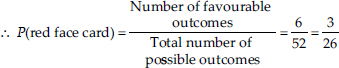(iv) Since, there is only 1 jack of hearts.
∴ Number of favourable outcomes = 1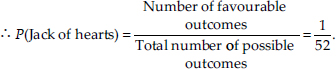(v) ∵ There are 13 spades in a pack of 52 cards:
∴ Favourable outcomes = 13.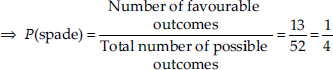(vi) ∵ There is only one queen of diamond.
∴ Number of favourable outcomes = 1
P(queen of diamonds)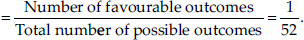Q 15.

Five cards-the ten, jack, queen, king and ace of diamonds, are well-shuffled with their face downwards. One card is then picked up at random.
(i) What is the probability that the card is the queen ?
(ii) If the queen is drawn and put aside, what is the probability that the second card picked up is (A) an ace? and (B) a queen?

SOLUTION:

Soln. : We have five cards.
∴ All possible outcomes = 5
(i) ∵ Number of queens = 1
∴ Number of favourable outcomes = 1
P(queen)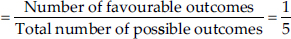(ii) The queen is drawn and put aside,
∴ Only 5 - 1 = 4 cards are left,
⇒ All possible outcomes = 4
(A) ∵ There is only one ace
∴ Number of favourable outcomes = 1
P(an ace)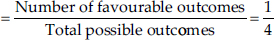(B) Since, the only queen has already been put aside.
∴ Number of possible outcomes = 0
P(a queen)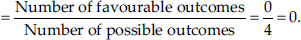Q 16.

12 defective pens are accidentally mixed with 132 good ones. It is not possible to just look at a pen and tell whether or not it is defective. One pen is taken out at random from this lot. Determine the probability that the pen taken out is a good one.

SOLUTION:

Soln. : We have number of good pens = 132 and number of defective pens = 12
∴ Total number of pens = 132 + 12 = 144 = Total possible outcomes.
There are 132 good pens
∴ Number of favourable outcomes = 132
P(good pens)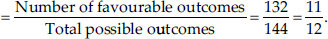Q 17.

(i) A lot of 20 bulbs contain 4 defective bulbs. One bulb is drawn at random from the lot. What is the probability that this bulb is defective?
(ii) Suppose the bulb drawn in (i) is not defective and is not replaced. Now one bulb is drawn at random from the rest. What is the probability that this bulb is not defective?

SOLUTION:

Soln. : Since, there are 20 bulbs in the lot.
∴ Total number of possible outcomes = 20
(i) ∵ Number of defective bulbs = 4
∴ Favourable outcomes = 4
P(defective bulb)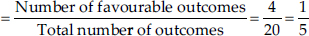(ii) ∵ The bulb drawn above is not included in the lot.
∴ Number of remaining bulbs = 20 - 1 = 19.
⇒ Total number of possible outcomes = 19.
∵ Number of bulbs which are not defective = 19 - 4 = 15
⇒ Favourable number of outcomes = 15
P(not defective bulb)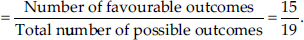Q 18.

A box contains 90 discs which are numbered from 1 to 90. If one disc is drawn at random from the box, find the probability that it bears (i) a two-digit number, (ii) a perfect square number and
(iii) a number divisible by 5.

SOLUTION:

Soln. : We have total number of discs = 90
∴ Total number of possible outcomes = 90
(i) Since the two-digit numbers are 10, 11,
12, ....., 90.
∴ Number of two-digit numbers = 90 - 9 = 81
⇒ Number of favourable outcomes = 81
P(Two digit number)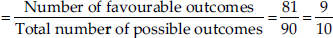(ii) Perfect square from 1 to 90 are 1, 4, 9, 16, 25, 36, 49, 64 and 81.
∴ Number of perfect squares = 9
⇒ Number of favourable outcomes = 9
P(Perfect square) =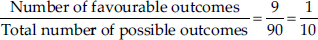(iii) Numbers divisible by 5 from 1 to 90 are 5, 10, 15, 20, 25, 30, 35, 40, 45, 50, 55, 60, 65, 70, 75, 80, 85, 90
i.e. There are 18 numbers from (1 to 90) which are divisible by 5.
∴ Numbers of favourable outcomes = 18
P(Number divisible by 5)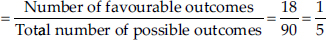Q 19.

A child has a die whose six faces shows the letters as given below: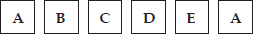The die is thrown once. What is the probability of getting (i) A? and (ii) D?

SOLUTION:

Soln. : Since there are six faces of the given die and these faces are marked with letters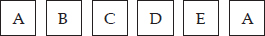∴ Total number of letters = 6
⇒ Number of possible outcomes = 6
(i) ∵ Two faces are having the letter A.
∴ Number of favourable outcomes = 2
Now, P(letter A)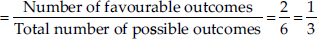(ii) ∵ Number of D' s = 1
∴ Number of possible outcomes = 1
P(letter D)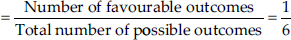Q 20.

Suppose you drop a die at random on the rectangular region shown in figure. What is the probability that it will land inside the circle with diameter 1 m?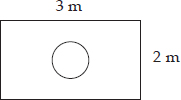SOLUTION:

Soln. : Here, area of the rectangle
= 3 m × 2 m = 6 m2
And, the area of the circle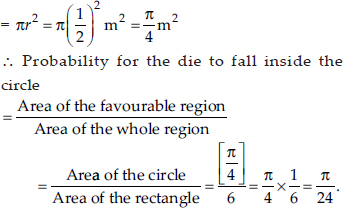Q 21.

A lot consists of 144 ball pens of which 20 are defective and the others are good. Nuri will buy a pen if it is good, but will not buy if it is defective. The shopkeeper draws one pen at random and gives it to her. What is the probability that
(ii) She will not buy it?

SOLUTION:

Soln. : Total number of ball pens = 144
⇒ Total number of possible outcomes = 144
(i) Since there are 20 defective pens
∴ Number of good pens = 144 - 20 = 124
⇒ Number of favourable outcomes = 124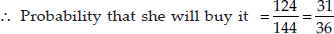(ii) Probability that she will not buy it
= 1 - [Probability that she will buy it]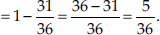Q 22.

Two dice, one blue and one grey, are thrown at the same time. Write all the possible outcomes. An event is defined as the sum of the two numbers appearing on the top of the dice.
(i) Complete the following table: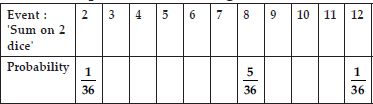(ii) A student argues that there are 11 possible outcomes 2, 3, 4, 5, 6, 7, 8, 9, 10, 11 and 12. Therefore, each of them has a probability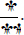Do you agree with his argument? Justify your answer.

SOLUTION:

Soln. : ∵ The two dice are thrown together.
∴ Following are the possible outcomes :
(1, 1) ; (1, 2); (1, 3); (1, 4); (1, 5); (1, 6).
(2, 1) ; (2, 2); (2, 3); (2, 4); (2, 5); (2, 6).
(3, 1) ; (3, 2); (3, 3); (3, 4); (3, 5); (3, 6).
(4, 1) ; (4, 2); (4, 3); (4, 4); (4, 5); (4, 6).
(5, 1) ; (5, 2); (5, 3); (5, 4); (5, 5); (5, 6).
(6, 1) ; (6, 2); (6, 3); (6, 4); (6, 5); (6, 6).
⇒ Number of all possible outcomes is 6 × 6 = 36
(i) (a) ∵ The sum on two dice is 3 for:
(1, 2) and (2, 1)
∴ Favourable outcomes = 2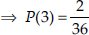(b) ∵ The sum on two dice is 4 for:
(1, 3), (2, 2) and (3, 1).
∴ Number of favourable outcomes = 3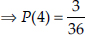(c) ∵ The sum on two dice is 5 for :
(1, 4), (2, 3), (3, 2) and (4, 1)
∴ Number of favourable outcomes = 4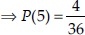(d) The sum on two dice is 6 for :
(1, 5), (2, 4), (3, 3), (4, 2) and (5, 1)
∴ Number of favourable outcomes = 5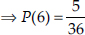(e) The sum on two dice is 7 for :
(1, 6), (2, 5), (3, 4), (4, 3), (5, 2) and (6, 1)
∴ Number of favourable outcomes = 6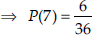(f) The sum on two dice is 9 for :
(3, 6), (4, 5), (5, 4) and (6, 3)
∴ Number of favourable outcomes = 4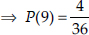(g) The sum on two dice is 10 for :
(4, 6), (5, 5), (6, 4)
∴ Number of favourable outcomes = 3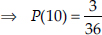(h) The sum on two dice is 11 for : (5, 6) and (6, 5)
∴ Number of favourable outcomes = 2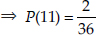Thus, the complete table is as under: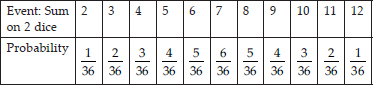(ii) No, the number of all possible outcomes is 36 not 11.
∴ The argument is not correct.

Q 23.

A game consists of tossing a one rupee coin 3 times and noting its outcomes each time. Hanif wins if all the tosses give the same result i.e., three heads or three tails, and loses otherwise. Calculate the probability that Hanif will lose the game.

SOLUTION:

Soln. : Let T denotes the tail and H denotes the head.
∴ All the possible outcomes are:
{H H H, H H T, H T T, T T T, T T H, T H T,
T H H, H T H}
∴ Number of all possible outcomes = 8
Let the event that Hanif will lose the game be denoted by E.
∴ Favourable events are:
{H H T, H T H, T H H, T H T, T T H, H T T}
⇒ Number of favourable outcomes = 6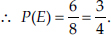Q 24.

A die is thrown twice. What is the probability that
(i) 5 will not come up either time? (ii) 5 will come up at least once?
[Hint: Throwing a die twice and throwing two dice simultaneously are treated as the same experiment.]

SOLUTION:

Soln. : Since, throwing a die twice or throwing two dice simultaneously is the same.
∴ All possible outcomes are:
(1, 1) ; (1, 2); (1, 3); (1, 4); (1, 5); (1, 6).
(2, 1) ; (2, 2); (2, 3); (2, 4); (2, 5); (2, 6).
(3, 1) ; (3, 2); (3, 3); (3, 4); (3, 5); (3, 6).
(4, 1) ; (4, 2); (4, 3); (4, 4); (4, 5); (4, 6).
(5, 1) ; (5, 2); (5, 3); (5, 4); (5, 5); (5, 6).
(6, 1) ; (6, 2); (6, 3); (6, 4); (6, 5); (6, 6).
∴ All possible outcomes = 36
(i) Let E be the event that 5 does not come up either time, then
The favourable outcomes are = [36 - (5 + 6)] = 25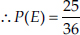(ii) Let N be the event that 5 will come up at least once, then number of favourable outcomes = 5 + 6 = 11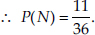Q 25.

Which of the following arguments are correct and which are not correct? Give reasons for your answer.
(i) If two coins are tossed simultaneously there are three possible outcomes two heads, two tails or one of each. Therefore, for each of these outcomes, the probability is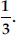(ii) If a die is thrown, there are two possible outcomes an odd number or an even number. Therefore, the probability of getting an odd number is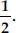SOLUTION:

Soln. : (i) Given argument is not correct. Because, if two coins are tossed simultaneously then four outcomes are possible (HH, HT, TH, TT). So total outcomes is 4.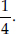(ii) Correct. Because the two outcomes are possible. Total outcomes = 6 and odd numbers = 3 and even numbers = 3.
So, favourable outcomes = 3 (in both the cases even or odd ).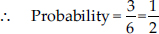Q 26.

Two customers Shyam and Ekta are visiting a particular shop in the same week (Tuesday to Saturday). Each is equally likely to visit the shop on any day as on another day. What is the probability that both will visit the shop on (i) the same day? (ii) consecutive days? (iii) different days?

SOLUTION:

Soln. : Here, the number of all the possible outcomes = 5 × 5 = 25
(i) For both customers visiting on same day:
Number of favourable outcomes = 5
[∵ (Tue., Tue.), (Wed., Wed.), (Thu., Thu.),
(Fri., Fri.), (Sat., Sat.)]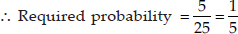(ii) For both the customers visiting on consecutive days:
Number of favourable outcomes:
(Tue., Wed.), (Wed., Thu.), (Thu., Fri.),
(Fri., Sat.), (Sat., Fri.), (Wed., Tue.),
(Thu., Wed.), (Fri., Thu.)
∴ Number of favourable outcomes = 8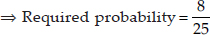(iii) For both the customers visiting on different days:
We have probability for both visiting on same day =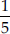∴ Probability for both visiting on different days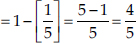⇒ The required probability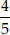Q 27.

A die is numbered in such a way that its faces show the numbers 1, 2, 2, 3, 3, 6. It is thrown two times and the total score in two throws is noted. Complete the following table which gives a few values of the total score in the two throws: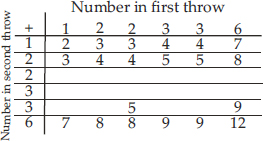What is the probability that the total score is
(i) even? (ii) 6? (iii) at least 6?

SOLUTION:

Soln. : The completed table is as under: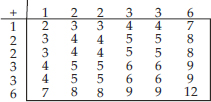∴ Number of all possible outcomes = 36
(i) For total score being even:
Favourable outcomes = 18
[∵ The even outcomes are: 2, 4, 4, 4, 4, 8, 4, 4, 8, 4, 6, 6, 4, 6, 6, 8, 8, 12]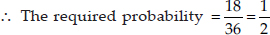(ii) For the scores being 6 :
In list of scores, we have four 6's.
∴ Favourable outcomes = 4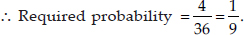(iii) For the score being at least 6:
The favourable scores are : 7, 8, 8, 6, 6, 9, 6, 6, 9, 7, 8, 8, 9, 9 and 12
∴ Number of favourable outcomes = 15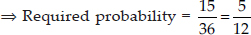Q 28.

A bag contains 5 red balls and some blue balls. If the probability of drawing a blue ball is double that of a red ball, determine the number of blue balls in the bag.

SOLUTION:

Soln. : Let the number of blue balls in the bag be x.
∴ Total number of balls = x + 5
Number of possible outcomes = (x + 5).
For a blue ball, favourable outcomes = x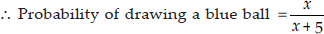Similarly, probability of drawing a red ball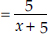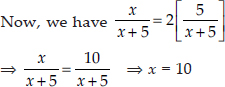Thus the required number of blue balls = 10.

Q 29.

A box contains 12 balls out of which x are black. If one ball is drawn at random from the box, what is the probability that it will be a black ball?
If 6 more black balls are put in the box, the probability of drawing a black ball is now double of what it was before. Find x.

SOLUTION:

Soln. : ∵ The total number of balls in the box = 12
∴ Number of possible outcomes = 12
Case-I: For drawing a black ball
Number of favourable outcomes = x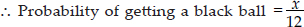Case-II: When 6 more black balls are added
Now, the total number of balls = 12 + 6 = 18
⇒ Number of possible outcomes = 18
Now, the number of black balls = (x + 6).
∴ Number of favourable outcomes = (x + 6)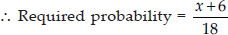Applying the given condition: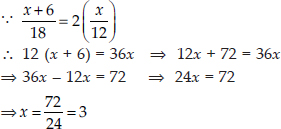Thus, the required value of x is 3.

Q 30.

A jar contains 24 marbles, some are green and others are blue. If a marble is drawn at random from the jar, the probability that it is green is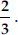Find the number of blue marbles in the jar.

SOLUTION:

Soln. : ∵ There are 24 marbles in the jar.
∴ Number of possible outcomes = 24.
Let there are x blue marbles in the jar.
∴ Number of green marbles = 24 - x
⇒ Favourable outcomes = (24 - x)
∴ Required probability for drawing a green marble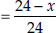Now, according to the condition, we have :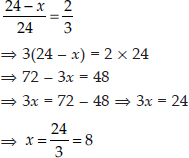Thus, the required number of blue marbles is 8.